# RD Sharma Solutions for Class 6 Chapter 1: Knowing Our Numbers Exercise 1.10

## RD Sharma Solutions for Class 6 Maths Exercise 1.10 PDF

The students are provided with exercise wise solutions to help them obtain a good score in the exam. The solutions are designed and well structured by the faculty at BYJU’S based on the latest syllabus of CBSE board. The problems are solved in an interactive manner to make the subject more interesting for the students. The students can use PDF of RD Sharma solutions while solving exercise wise problems which boosts confidence while appearing for the exam. RD Sharma Solutions for Class 6 Maths Chapter 1 Knowing Our Numbers Exercise 1.10 are provided here.

## RD Sharma Solutions for Class 6 Chapter 1: Knowing Our Numbers Exercise 1.10 Download PDF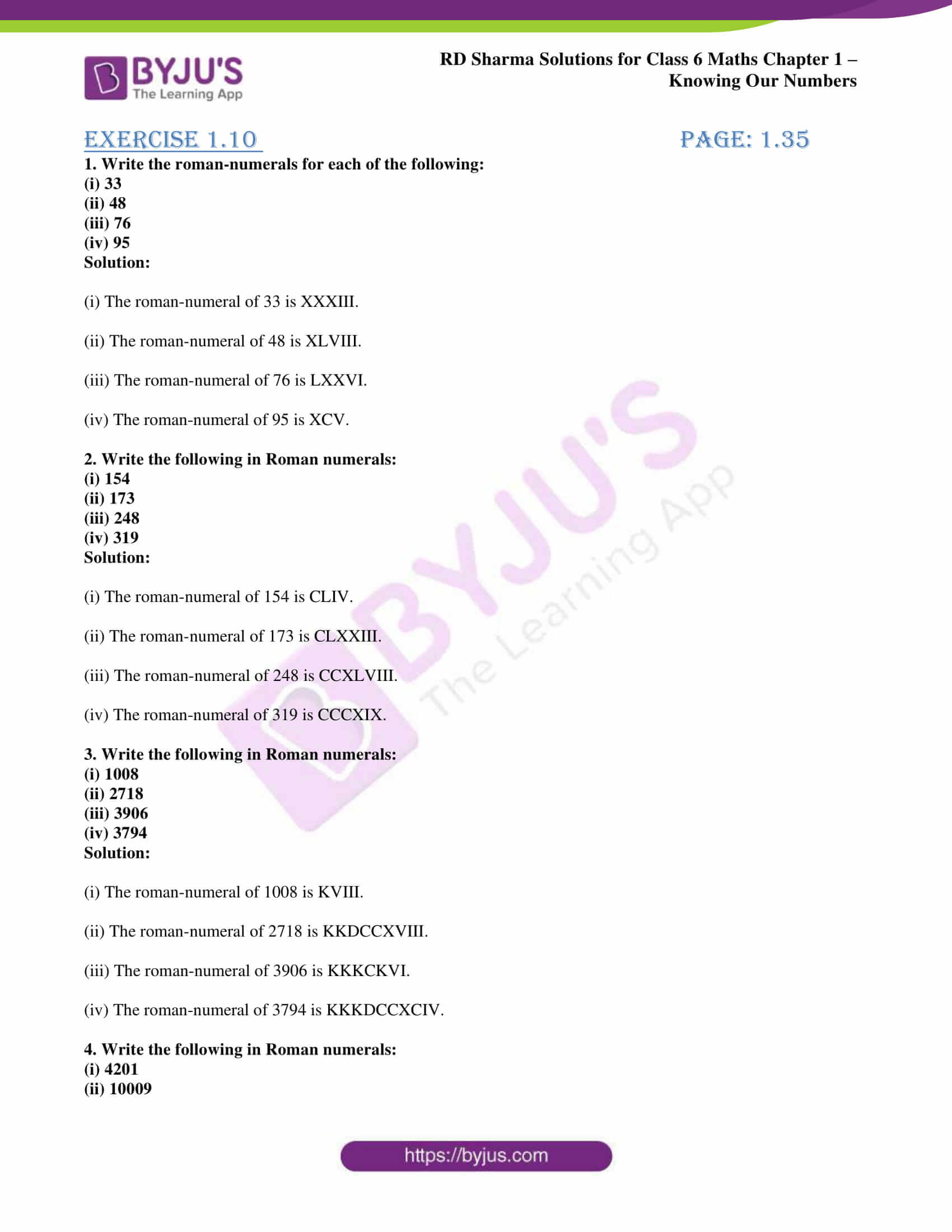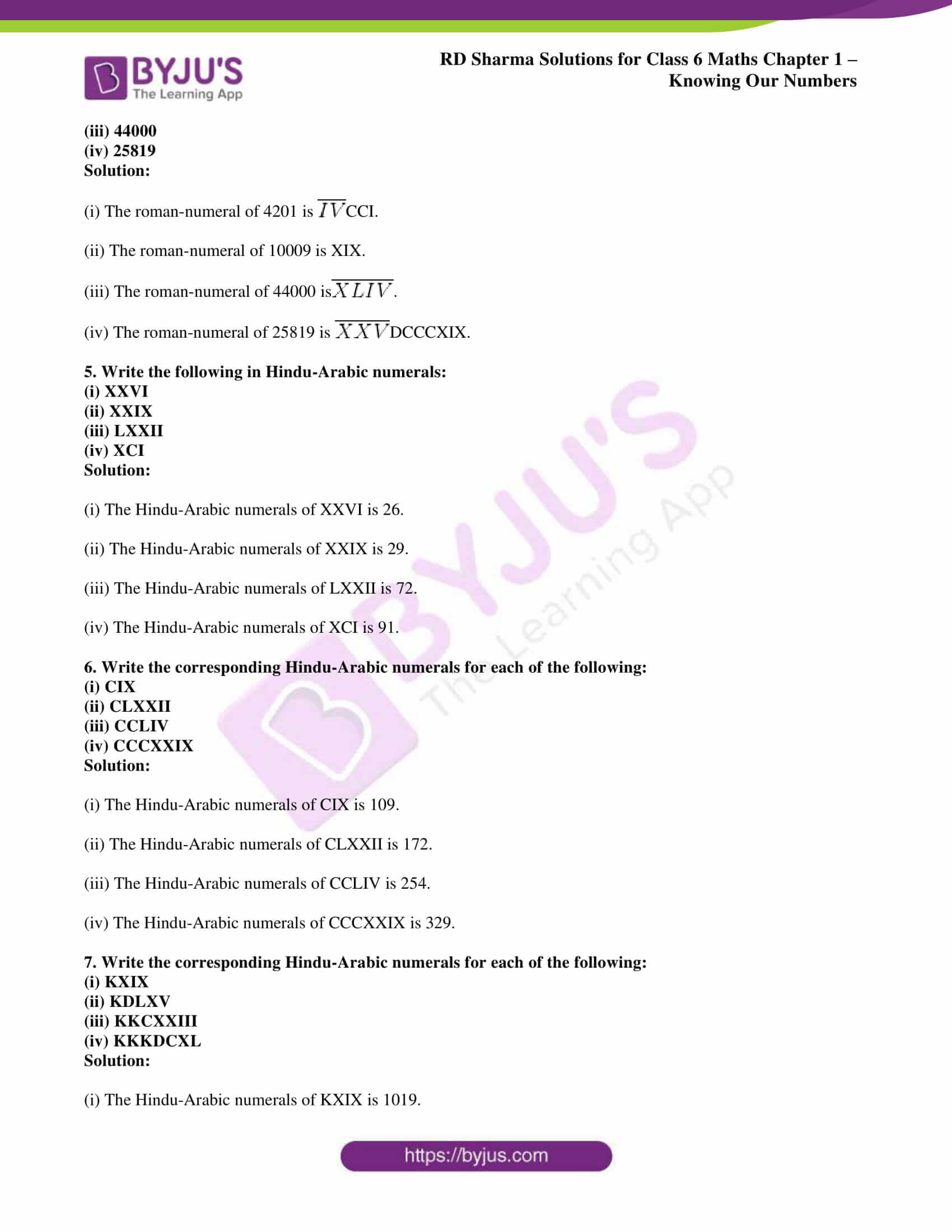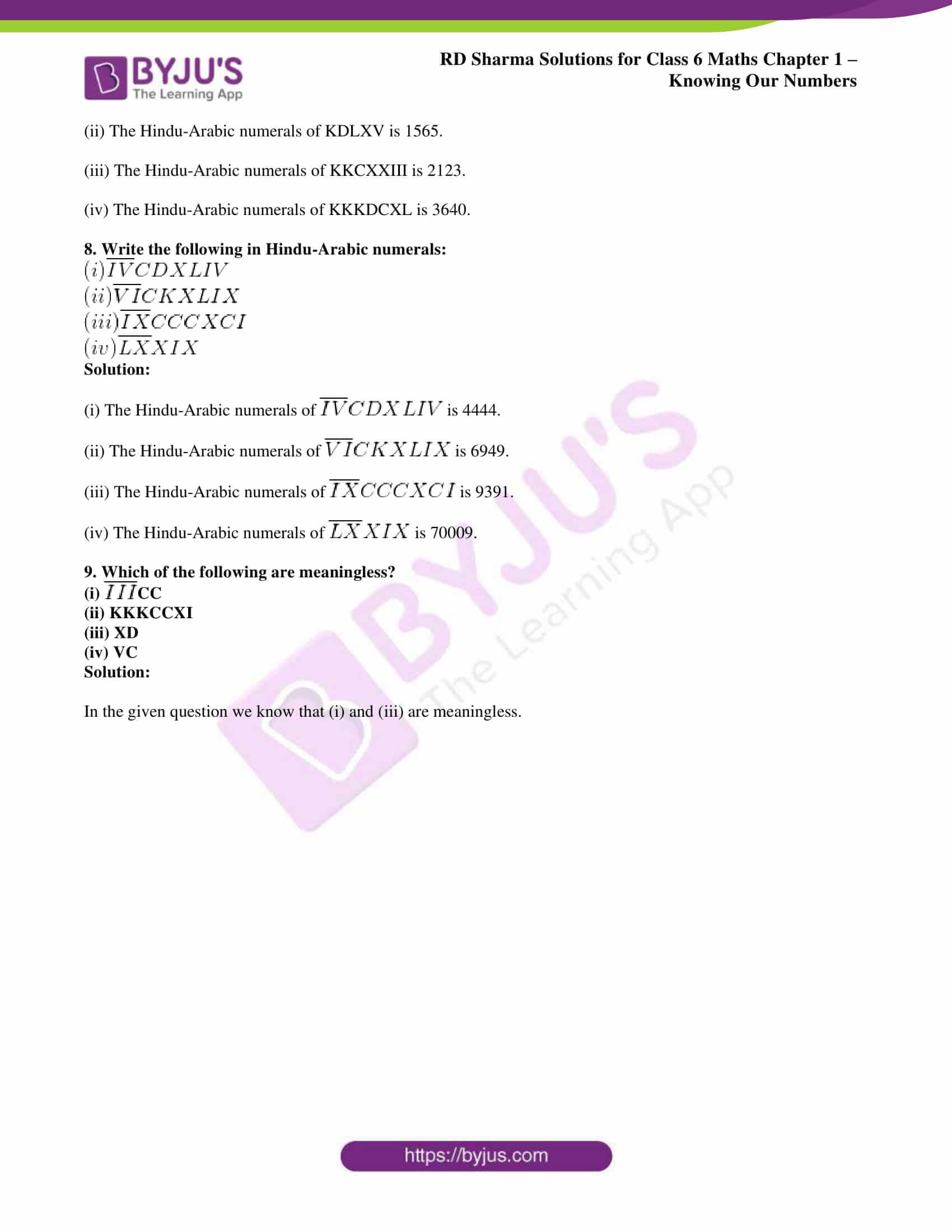## Access RD Sharma Solutions for Class 6 Chapter 1: Knowing Our Numbers Exercise 1.10

1. Write the roman-numerals for each of the following:

(i) 33

(ii) 48

(iii) 76

(iv) 95

Solution:

(i) The roman-numeral of 33 is XXXIII.

(ii) The roman-numeral of 48 is XLVIII.

(iii) The roman-numeral of 76 is LXXVI.

(iv) The roman-numeral of 95 is XCV.

2. Write the following in Roman numerals:

(i) 154

(ii) 173

(iii) 248

(iv) 319

Solution:

(i) The roman-numeral of 154 is CLIV.

(ii) The roman-numeral of 173 is CLXXIII.

(iii) The roman-numeral of 248 is CCXLVIII.

(iv) The roman-numeral of 319 is CCCXIX.

3. Write the following in Roman numerals:

(i) 1008

(ii) 2718

(iii) 3906

(iv) 3794

Solution:

(i) The roman-numeral of 1008 is KVIII.

(ii) The roman-numeral of 2718 is KKDCCXVIII.

(iii) The roman-numeral of 3906 is KKKCKVI.

(iv) The roman-numeral of 3794 is KKKDCCXCIV.

4. Write the following in Roman numerals:

(i) 4201

(ii) 10009

(iii) 44000

(iv) 25819

Solution:

(i) The roman-numeral of 4201 is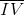CCI.

(ii) The roman-numeral of 10009 is XIX.

(iii) The roman-numeral of 44000 is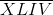.

(iv) The roman-numeral of 25819 is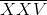DCCCXIX.

5. Write the following in Hindu-Arabic numerals:

(i) XXVI

(ii) XXIX

(iii) LXXII

(iv) XCI

Solution:

(i) The Hindu-Arabic numerals of XXVI is 26.

(ii) The Hindu-Arabic numerals of XXIX is 29.

(iii) The Hindu-Arabic numerals of LXXII is 72.

(iv) The Hindu-Arabic numerals of XCI is 91.

6. Write the corresponding Hindu-Arabic numerals for each of the following:

(i) CIX

(ii) CLXXII

(iii) CCLIV

(iv) CCCXXIX

Solution:

(i) The Hindu-Arabic numerals of CIX is 109.

(ii) The Hindu-Arabic numerals of CLXXII is 172.

(iii) The Hindu-Arabic numerals of CCLIV is 254.

(iv) The Hindu-Arabic numerals of CCCXXIX is 329.

7. Write the corresponding Hindu-Arabic numerals for each of the following:

(i) KXIX

(ii) KDLXV

(iii) KKCXXIII

(iv) KKKDCXL

Solution:

(i) The Hindu-Arabic numerals of KXIX is 1019.

(ii) The Hindu-Arabic numerals of KDLXV is 1565.

(iii) The Hindu-Arabic numerals of KKCXXIII is 2123.

(iv) The Hindu-Arabic numerals of KKKDCXL is 3640.

8. Write the following in Hindu-Arabic numerals: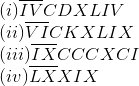Solution:

(i) The Hindu-Arabic numerals of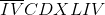is 4444.

(ii) The Hindu-Arabic numerals of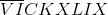is 6949.

(iii) The Hindu-Arabic numerals of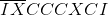is 9391.

(iv) The Hindu-Arabic numerals of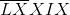is 70009.

9. Which of the following are meaningless?

(i)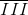CC

(ii) KKKCCXI

(iii) XD

(iv) VC

Solution:

In the given question we know that (i) and (iii) are meaningless.

### RD Sharma Solutions Class 6 Maths Chapter 1 – Knowing Our Numbers Exercise 1.10

RD Sharma Solutions Class 6 Maths Chapter 1 Knowing Our Numbers Exercise 1.10 is the tenth exercise which explains the method of writing Roman Numerals and Hindu-Arabic numerals for the exercise problems.

### Key features of RD Sharma Solutions for Class 6 Maths Chapter 1: Knowing Our Numbers Exercise 1.10

• The methods used in solving exercise wise problems are explained in an interactive manner to make them easy for the students.
• Conceptual knowledge before solving exercise wise problems is improved with the help of RD Sharma solutions.
• The problems are solved by expert set of faculty having wide experience in the respective subject.